### Vector Calculus

In a nutshell, vector calculus deals with functions that output vectors.

For example, a wind map takes a location $P=(x,y)$ as input and returns the wind velocity vector $\vec{V}(x,y),$ displaying it as an arrow at $P.$

The wind map plots the vector function $\vec{V}(x,y).$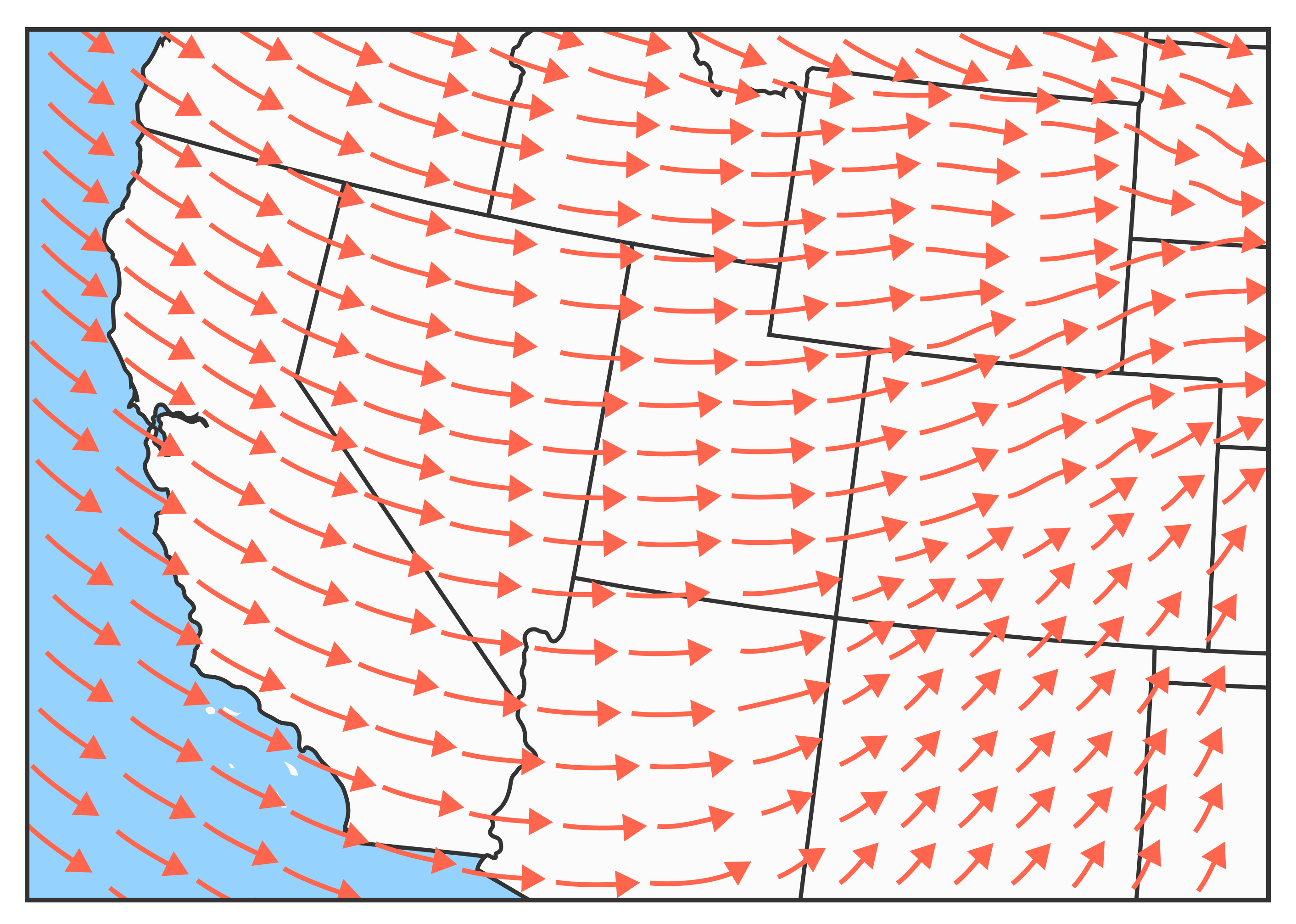# Vector Calculus in a Nutshell

The most important object in our course is the vector field, which assigns a vector to every point in some subset of space.

We'll cover the essential calculus of such vector functions, and explore how to use them to solve problems in partial differential equations, wave mechanics, electricity and magnetism, and much more!

This quiz kicks off a short intro to the essential ideas of vector calculus.

# Vector Calculus in a Nutshell

The gradient $\nabla f = \left \langle \frac{\partial f}{\partial x} , \frac{\partial f}{\partial y}, \frac{\partial f}{\partial z} \right \rangle$ provides an example familiar from multivariable calculus with many important properties.

The touch interactive plot below shows a level set $f = c$ of $f = \sqrt{x^2+ y^2+z^2}$ together with the gradient vector field $\nabla f.$

Zoom in and out and rotate to explore this 3D vector field, then select the true statements about $\nabla f$ from the options provided.

# Vector Calculus in a Nutshell

Select one or more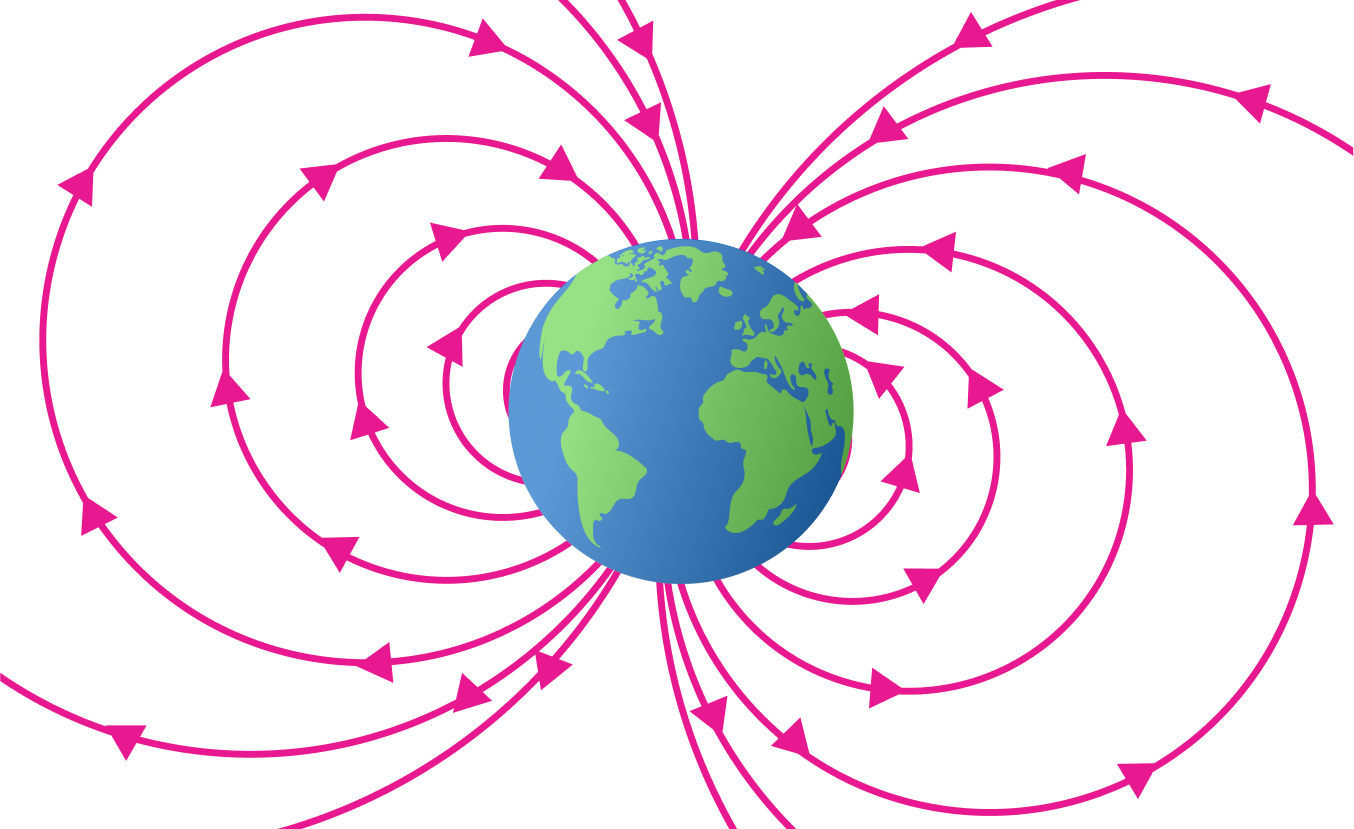If you've ever held a compass or seen the northern or southern lights, you're aware of Earth's natural magnetism.

The temperature of a cup of coffee, the flow of a river, and the amount of air in a child's balloon are probably more familiar to you from day-to-day experience.

Some are vector fields; others (the scalars) lack directionality and just have magnitude.

Select from the options all quantities that can also be modeled by a vector field.

# Vector Calculus in a Nutshell

Select one or more

To graph a vector field, we draw the arrow for $\vec{V}(\vec{x})$ with base at $\vec{x}$ for each point on a chosen grid, as in last problem's interactive plot. $\\\\$

Flow lines (aka field lines) also help us see important vector field features. A flow line is a curve that “follows the arrows”; if we're on the flow line at $\vec{x},$ $\vec{V}(\vec{x})$ points the way to our next stop along the curve.

We include a two-dimensional example and a three-dimensional one below.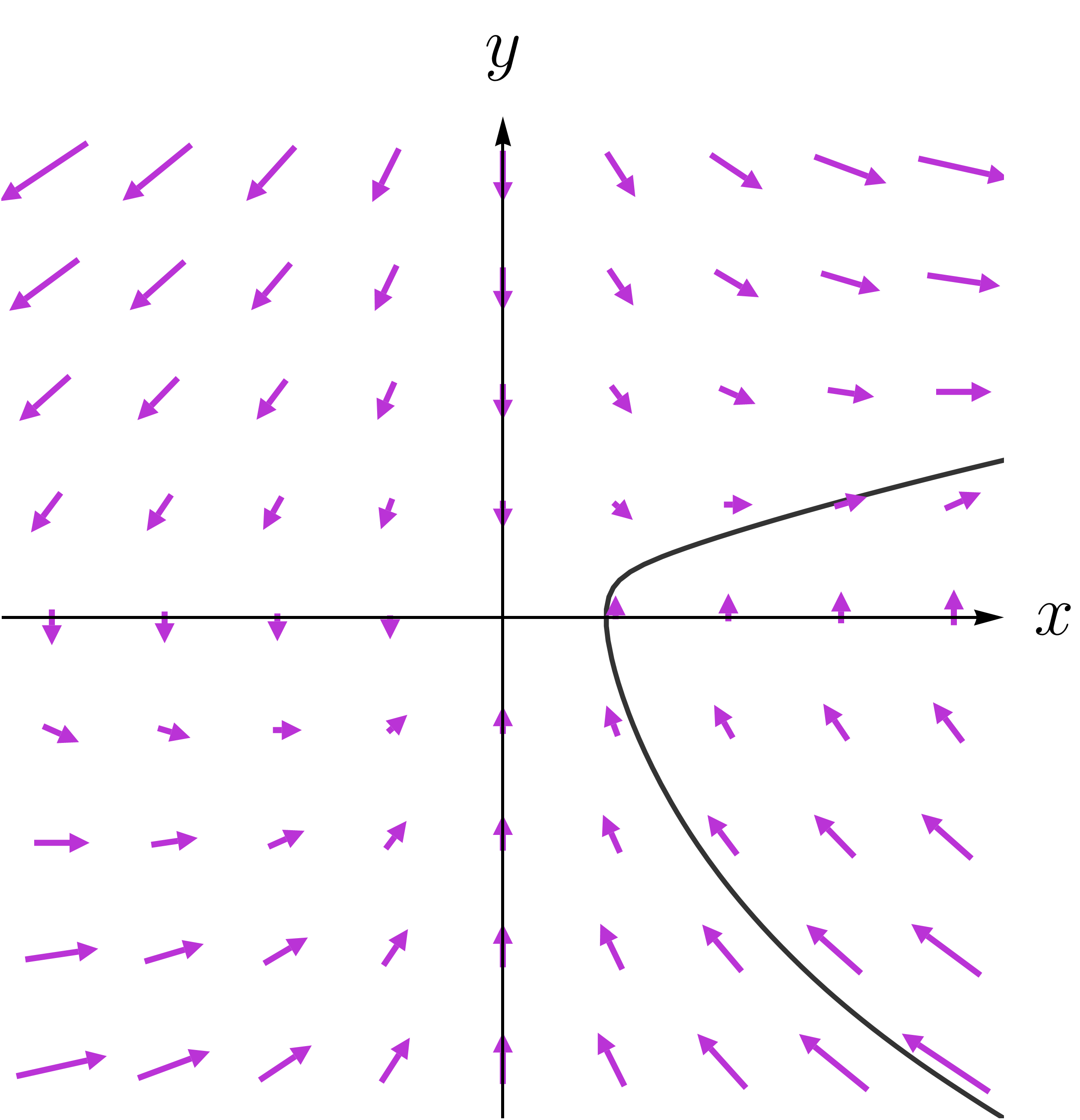$\\\\$

# Vector Calculus in a Nutshell

Using this intuitive notion of a flow line, choose the option that best describes a flow line for the two-dimensional vector field below.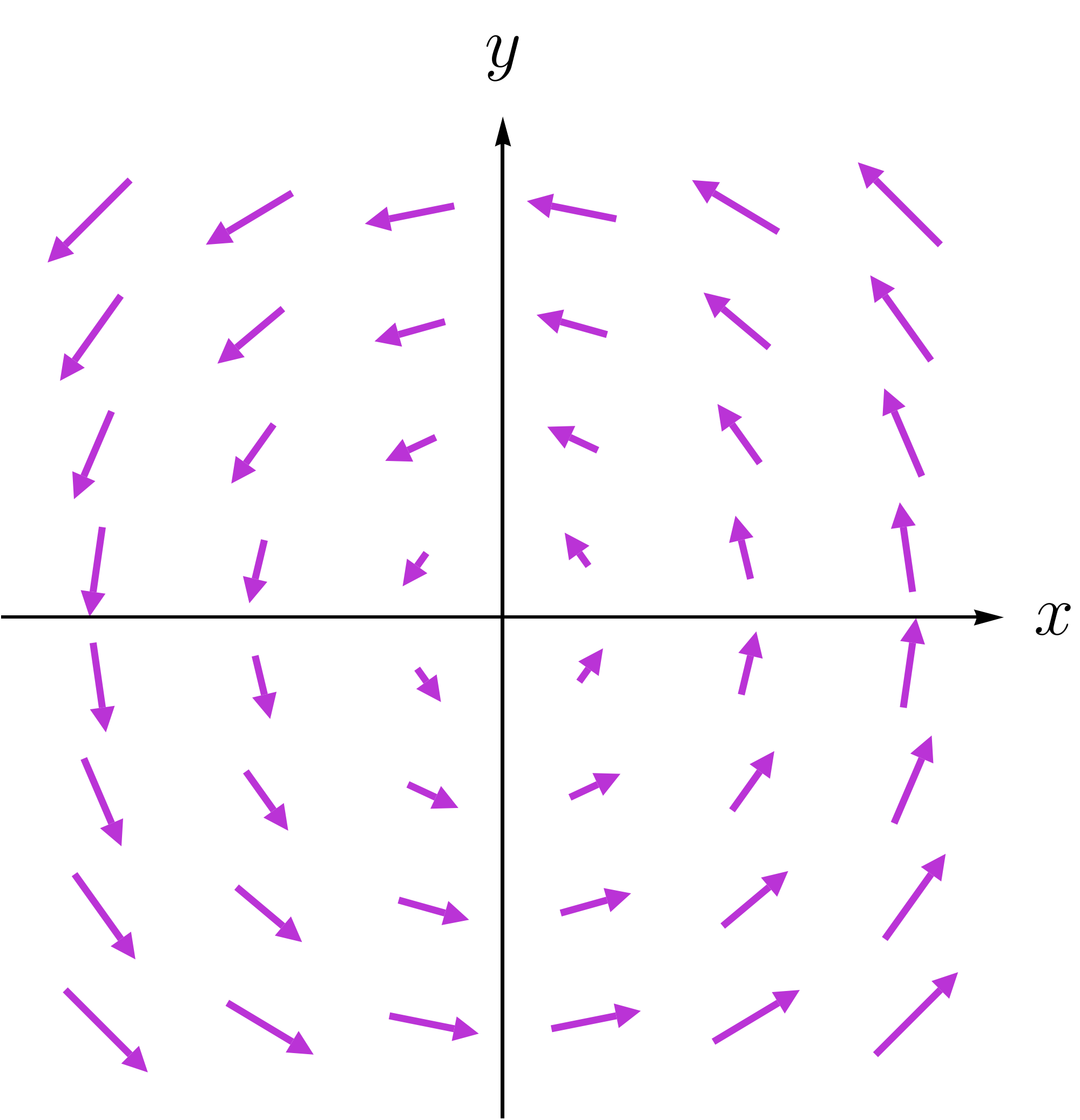# Vector Calculus in a Nutshell

We can use flow lines as a means of understanding one of the most important vector field derivatives: the divergence.

The animation shows what happens to a rectangle when every point in it flows along the vector field.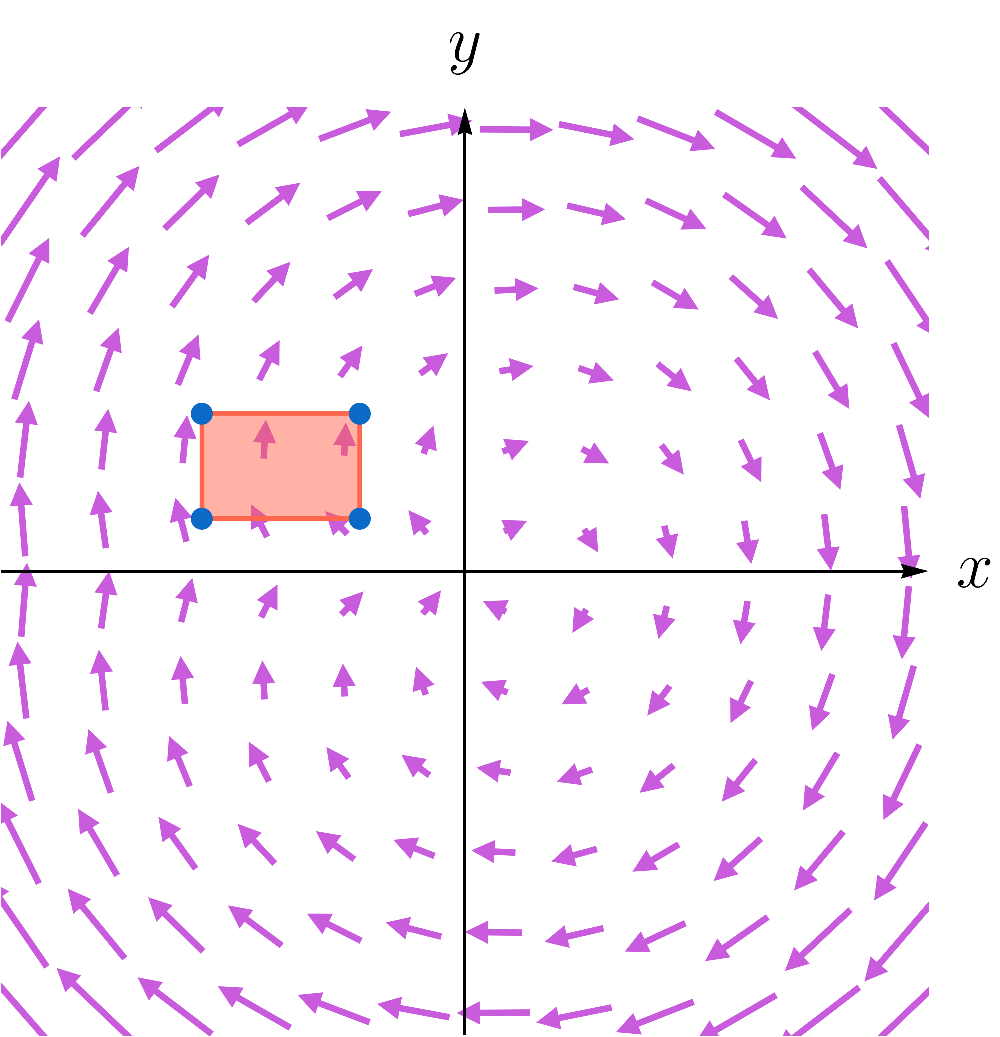It turns out that how much this rectangle distorts under flow is directly related to the divergence, which we'll talk about next.

# Vector Calculus in a Nutshell

As the name suggests, the divergence measures how much a vector field spreads out from a given point. We'll cover divergence in detail later in the course, but this qualitative description will do for right now.

Below are two vector fields. Select the one that has the greatest divergence from the origin.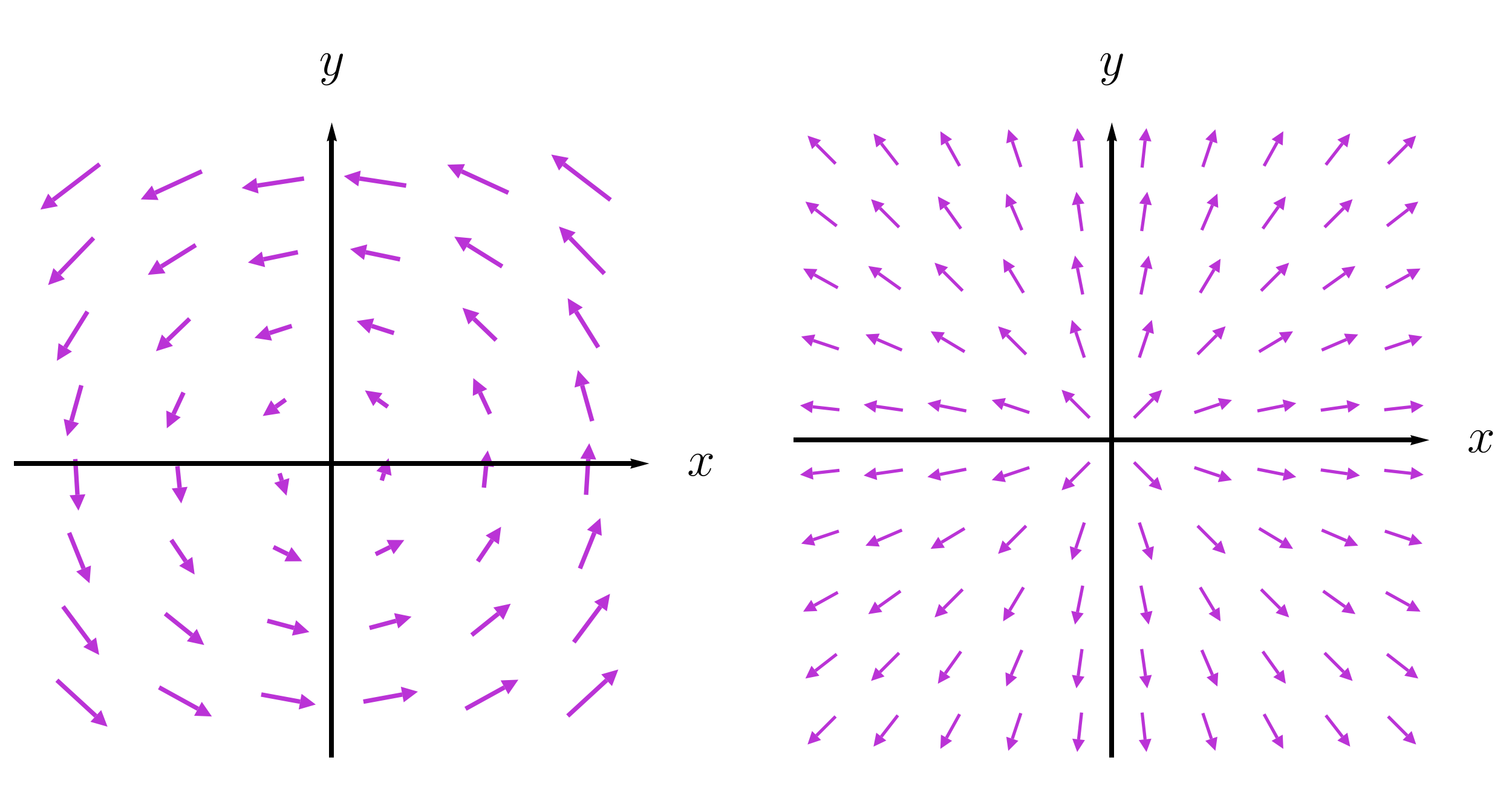Option I (left), Option II (right)

# Vector Calculus in a Nutshell

It'll take some work with flow lines to prove this, but the divergence of a two-dimensional vector field $\vec{V}(x,y) = \big\langle V_{x}(x,y) , V_{y}(x,y) \big\rangle$ at $(x,y)$ is $\text{div}\big(\vec{V}\big) = \frac{\partial V_{x}}{\partial x} + \frac{\partial V_{y}}{\partial y}.$ Option I in the previous problem (which just circled the origin) is explicitly given by $\langle -y, x \rangle$ and so has divergence $\text{div}\big( \langle -y, x \rangle \big) = \frac{\partial }{\partial x}[-y] + \frac{\partial}{\partial y}[x]=0.$

Compute the divergence for the vector field of option II given by $\vec{V}(x,y) = \left \langle x , y \right \rangle.$

# Vector Calculus in a Nutshell

The divergence formula comes from a careful analysis of flow lines, which we'll do later in the course. These curves also teach us much about another very important derivative called curl.

The flow of a river is like a 2D vector field; the strength and direction of current at a point is assigned an arrow there.

If we dump a few X-shaped paddle wheels into the stream, they'll move with the flow and also rotate as fluid strikes the paddles.

Each X is assigned a vector (called the curl) pointing along the $z$-axis and providing the axis of rotation. Its length is related to the spin rate. If it points up (down), the X spins counterclockwise (clockwise).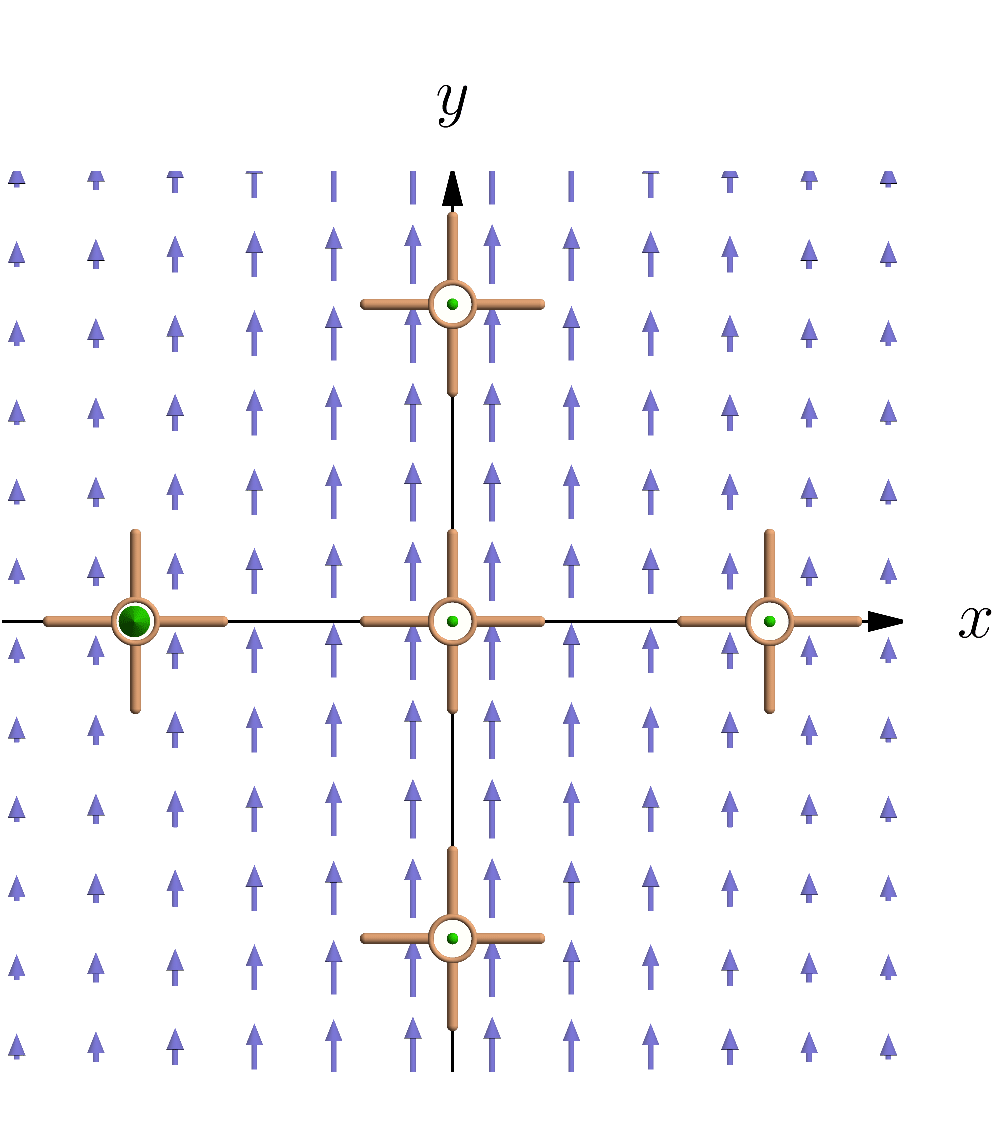# Vector Calculus in a Nutshell

The curl of a two-dimensional vector field is given by $\text{curl}\big(\vec{V}\big)(x,y) = \left[ \frac{\partial V_{y}}{\partial x} - \frac{\partial V_{x}}{\partial y} \right] \hat{k},$ as we'll see in greater detail later in the course.

Compute the curl of the vector field $\langle -y , x \rangle$ that we encountered earlier in this quiz.# Vector Calculus in a Nutshell

Just as divergence and curl provide two different vector field derivatives, line integrals and surface integrals give us different ways of integrating fields.

Vector integrals are a bit more complicated than vector derivatives, so we'll postpone talking about them for now.

In the next chapter, we'll make a detour into the world of motion and see some other applications of vector derivatives.

# Vector Calculus in a Nutshell

×